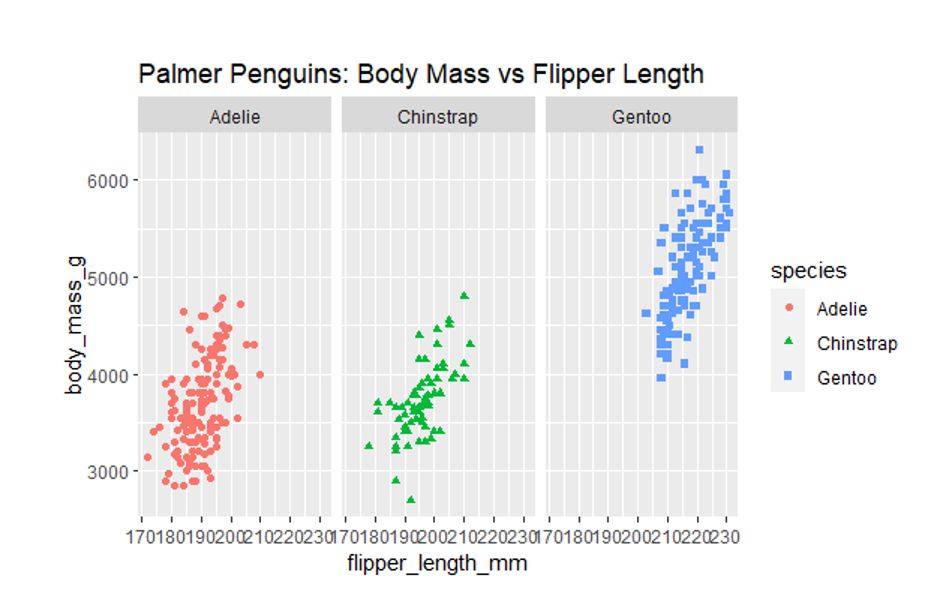# Scatter Plot using R

R is a programming language used for statistical analysis, visualization, and other data analysis. As a data analyst, I will use R to complete many of the tasks associated with the data analysis process. If you are not familiar with R programming language, don’t be worry because this is my first chart using R and I want to document it for me. I made it while following the course Google Data Analytics. Maybe, step by step making this chart can help you learn R.

Now, I want to make a scatterplot using R. A scatterplot is a chart to shows the relationship between two variables. In this case, I want to visualize the relationship between body mass and flipper length. We can guess the larger the penguin, the longer the flipper, right? :D.Fig 1. Relationship between body mass and flipper length (Created by Author)

2. Open RStudio.

I use two libraries so I need to install both, palmerpenguins and ggplot2. If you don’t know what a library is, a library is basically a collection of data, functions or code that has been created by programmers and we just have to use them.

Palmer penguins dataset contains size measurements for three penguin species that live on the Palmer Archipelago in Antarctica. This includes data on stuff like body mass, flipper length and bill length. The dataset has 344 rows of information sorted into eight columns.

3. Let’s code!

We have to call the library first so we can use it to visualize our data, so I code library palmerpenguins and ggplot2. I think the process of creating the graph is simple but the process of understanding the syntax at first may be confusing. If there is a term you don’t understand, please look it up on the internet.

• Code 1

Creating a plot > output just blank plot. So we need add some more code

• Code 2

Body mass is on the y-axis and flipper length is on the x-axis, but the data points are not yet visible.

• Code 3

To get the complete plot, we can add some more code that tells R how to represent our data. Use geom_points to scatter plot, dot plots,etc.

• Code 4

We can go further. I want to can change how the plot looks. I want change the color of all of the points to green. Then I add in color equals green inside geom point.

• Code 5

I can also add new information to the plot and use color to highlight it. Let’s tell R to assign a different color to each species of penguin. This way we can link data points to each group of penguins.

• Code 6

Gentoos are the largest. The legend just to the right of the plot shows us that the blue points refer to the Gentoos. R automatically creates a legend for the plot to help us understand the color-coding.

• Code 7

We can also use shape to highlight the different penguin species. Or we can use both color and shape. In addition to highlighting our data, we can also reorganize it. We can break our data down into smaller groups or subsets and create a plot for each subset. Let’s say I want to focus on the data for each species. Facet functions let us create a separate plot for each species.